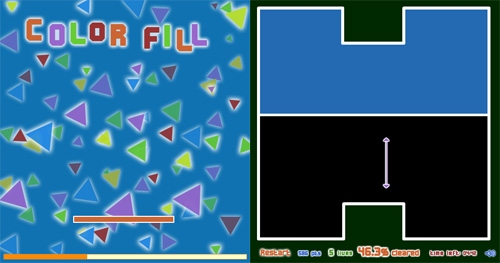# Create a Flash game like ColorFill – part 1

If you play all successful Flash games (and you should, if you want to be a Flash game developer), surely you played ColorFill.In this game, you have to fill 80% of the stage with colors while avoiding collisions with enemies.

Play the game a bit then follow this prototype

The main idea to create this game is based upon the “vertical state” of arrows. I designed the arrows as horizontal ones, but if the player presses SPACE, I rotate them by 90 degrees so the left one becomes the up one and the right one becomes the down one.

There’s not any more difficulty in this first part, when I still don’t fill the area once I successfully draw a line.

Some previous concepts from Create a flash draw game like Line Rider or others – part 2 are involved in order to determine collisions between the ball and the line.

```Mouse.hide();
// flag indicating if I pressed space
space_pressed = false;
// vertical_arrow = true: arrows are horizontal
// vertical_arrow = false: arrows are vertical
vertical_arrow = false;
// flag indicating if I pressed space
clicked = false;
// enemy's random angle movement
angle = Math.random()*360;
// enemy and arrows speeds
speed = 5;
// booleans that states if the left or right arrows touched the border of the stage
left_touch = false;
right_touch = false;
// x and y speeds of the enemy
xspeed = speed*Math.cos(angle*0.0174532925);
yspeed = speed*Math.sin(angle*0.0174532925);
// enemy collision detection precision
precision = 16;
// attaching the ball = the enemy
_root.attachMovie("ball","ball",1,{_x:250, _y:200});
// creating the empty movieclip that will contain all drawings
_root.createEmptyMovieClip("line",2);
// attaching the left arrow
_root.attachMovie("left_arrow","left_arrow",3);
// attaching the right arrow
_root.attachMovie("right_arrow","right_arrow",4);
// function to be executed for the left arrow
left_arrow.onEnterFrame = function() {
// if I did not click the mouse
if (!clicked) {
// if I am not in "vertical mode"...
if (!vertical_arrow) {
// place the arrow
this._y = _root._ymouse;
this._x = _root._xmouse-15;
this._rotation = 0;
}
// if I am in "vertical mode"....
else {
// place the arrow
this._y = _root._ymouse-15;
this._x = _root._xmouse;
this._rotation = 90;
}
}
// if I clicked the mouse....
else {
// if the arrow did not touch the edge of the stage...
if (!left_touch) {
// if I am not in "vertical mode"...
if (!vertical_arrow) {
// moving the arrow
this._x -= speed;
// checking if the arrow touched the left edge of the stage
if (this._x<8) {
this._x = 8;
left_touch = true;
}
}
// if I am in "vertical mode"...
else {
// moving the arrow
this._y -= speed;
// checking if the arrow touched the upper edge of the stage
if (this._y<8) {
this._y = 8;
left_touch = true;
}
}
}
}
};
// same concept for the right arrow
right_arrow.onEnterFrame = function() {
if (!clicked) {
if (!vertical_arrow) {
this._y = _root._ymouse;
this._x = _root._xmouse+15;
this._rotation = 0;
}
else {
this._y = _root._ymouse+15;
this._x = _root._xmouse;
this._rotation = 90;
}
}
else {
if (!right_touch) {
if (!vertical_arrow) {
this._x += speed;
if (this._x>492) {
this._x = 492;
right_touch = true;
}
}
else {
this._y += speed;
if (this._y>392) {
this._y = 392;
right_touch = true;
}
}
}
}
};
// function to be executed for the ball... it's a simple "bounce here and there"
ball.onEnterFrame = function() {
this._x += xspeed;
this._y += yspeed;
if ((this._x<20) or (this._x>480)) {
xspeed *= -1;
}
if ((this._y<20) or (this._y>380)) {
yspeed *= -1;
}
};
// function to be executed at every frame
_root.onEnterFrame = function() {
// if I did not pressed the space....
if (!space_pressed) {
// checking if I am pressing the space
if (Key.isDown(Key.SPACE)) {
space_pressed = true;
// switching vertical_arrow state between true and false
vertical_arrow = !vertical_arrow;
}
}
// if I pressed space...
else {
// checking if I released the space
if (!Key.isDown(Key.SPACE)) {
space_pressed = false;
}
}
// checking if I clicked the mouse
if (clicked) {
// preparing the line style
line.clear();
line.lineStyle(5,"0xff0000");
// drawing a line from an arrow to the other
line.moveTo(left_arrow._x,left_arrow._y);
line.lineTo(right_arrow._x,right_arrow._y);
// checking collision between the ball and the line
for (x=1; x
And this is the result:

Now next challenge is painting the area just closed with the line and manage new stage bounds.
```
214 GAME PROTOTYPES EXPLAINED WITH SOURCE CODE
// 1+2=3
// 10000000
// 2 Cars
// 2048
// Avoider
// Ballz
// Block it
// Blockage
// Bloons
// Boids
// Bombuzal
// Breakout
// Bricks
// Columns
// CubesOut
// Dots
// DROP'd
// Dudeski
// Eskiv
// Filler
// Fling
// Globe
// HookPod
// Hundreds
// InkTd
// Iromeku
// Lumines
// Magick
// MagOrMin
// Maze
// Memdot
// Nano War
// Nodes
// o:anquan
// Ononmin
// Pacco
// Phyballs
// Platform
// Poker
// Pool
// Poux
// Pudi
// qomp
// Racing
// Renju
// SameGame
// Security
// Sling
// Slingy
// Sokoban
// Splitter
// Sproing
// Stack
// Stringy
// Sudoku
// Tetris
// Threes
// Toony
// Turn
// TwinSpin
// vvvvvv
// Wordle
// Worms
// Yanga
// Zhed
// zNumbers